### 14.24. Line Nova

#### 14.24.1. Overview

Figure 17.370. Example for the Line Nova filterOriginal image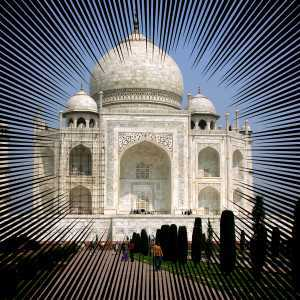Line Nova applied

The Line Nova filter fills a layer with rays emanating outward from the center of the layer using the foreground color shown in the Toolbox. The rays starts as one pixel and grew broader towards the edges of the layer.

Tip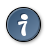This filter does not provide any option which allows you to set the center point of lines. If you need adjust the place of the radial lines where you want, create another transparent image and apply this filter on it, then add it on your image. Setting large size for the new nova image may help you not to break lines inside of your image.

#### 14.24.2. Activating the filter

This filter is found in the image window menu under FiltersRenderLine Nova….

#### 14.24.3. Options

Figure 17.371. Line Nova options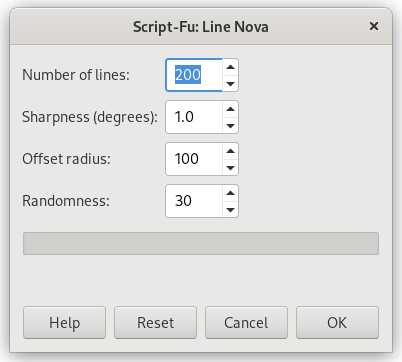Number of lines

By using this option you can set the number of lines between 40 to 1000. The default is 200.

Sharpness (degrees)

This slider determines how much the rays will broaden towards the edges. The range goes from 0.0 to 10.0. If set to 0.0, nothing will be drawn. If set to 10.0, most of the area near the edges of the layer will be painted.

Figure 17.372. Line Nova sharpness option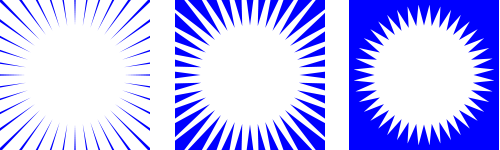From left to right: sharpness = 1; sharpness = 5; sharpness = 10

Here you choose the distance, in pixels, from center to the starting point of the rays. If set to 0.0 the rays starts from the center. Any other value will let the starting points be on a circle at the selected distance from the center. The maximum distance is 2000 pixels. The default value is 100 pixels.

Figure 17.373. Line Nova offset radius option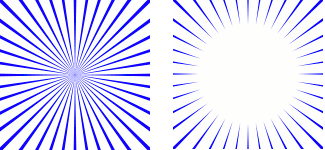From left to right: offset radius = 0; offset radius = 50

Randomness

If this slider is set to a value higher than 1, the starting point for each ray differ more or less randomly from the average starting point set as the offset radius above. With the value set to 1, all the rays will start at the circle determined by the offset radius. The maximum value is 2000. The default value is 30.

Figure 17.374. Line Nova randomness optionFrom left to right: randomness = 1; randomness = 50